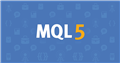# Update the highest price393

Hi, I have a question.

I want to record the current profit and if the profit is more than current profit, then I want to update the price.

Current \$10

5min later, it changed to \$20,

So I want to monitor this value.

``` static double MaxProfit;

if(MaxProfit<ProfitCheck() ){MaxProfit=ProfitCheck();}```

Wrong?

Sorry , what I want is

I want to show the current price (only plus profit), later the price is moved up, then I want to show that value.

For example,

The showing price is

\$10

\$12

After that

\$15........

etc....Documentation on MQL5: Constants, Enumerations and Structures / Trade Constants / Position Properties
• www.mql5.com
Position ticket. Unique number assigned to each newly opened position. It usually matches the ticket of an order used to open the position except when the ticket is changed as a result of service operations on the server, for example, when charging swaps with position re-opening. To find an order used to open a position, apply the...24704

Cromo:I want to record the current profit and if the profit is more than current profit, then I want to update the price.
1. Profit is money. There is no price.
2. State a problem.393

William Roeder:
1. Profit is money. There is no price.
2. State a problem.

Hi, William san

This is for profit count.

```    double ProfitCheck()
{
double Current_profit=0;
int total  = OrdersTotal();
for (int cnt = total-1 ; cnt >=0 ; cnt--){

OrderSelect(cnt, SELECT_BY_POS, MODE_TRADES);
Current_profit+=OrderProfit()+ OrderSwap() + OrderCommission();

}
return(Current_profit);
}
```

This profit is always changing.

I want to show this price always highest value.

Do you know what I mean?

For example, If the price becomes \$20  --- drop down ----- \$12....... again going up to \$19..........\$ 10 ,.......\$8.....

I want to print \$20. (Max value)24704

You already posted code that remembers the max. State a problem.393

William Roeder:
You already posted code that remembers the max. State a problem.

Yes, I want to Print (comment) the max profit value.

```static double MaxProfit;

if(MaxProfit<ProfitCheck() ){MaxProfit=ProfitCheck();}```

If I print (MaxProfit), the price is not showing the max value. How can I fix it?